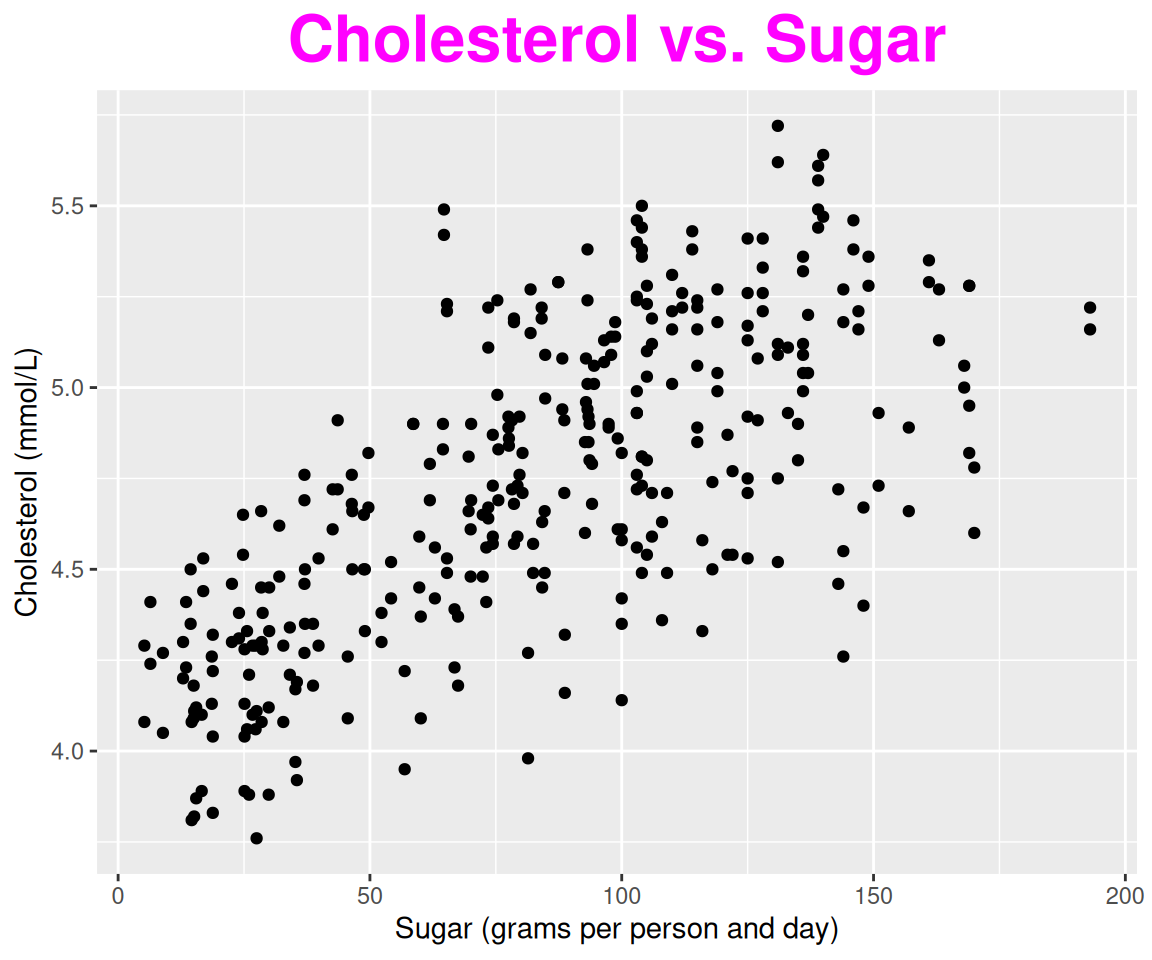## 4.15 Violin Plots

In this section, we introduce how to combine music with R via creating violin plots. In fact, the violin plot elegantly combines boxplot (Section 4.14) and density plots (Section 4.13) into a single plot.

### 4.15.1 The basic violin plot

Let’s say we want to generate a basic violin plot for the variable sale_price in the sahp dataset.

library(r02pro)
library(ggplot2)
ggplot(data = sahp,
aes(x = "", y = sale_price)) +
geom_violin()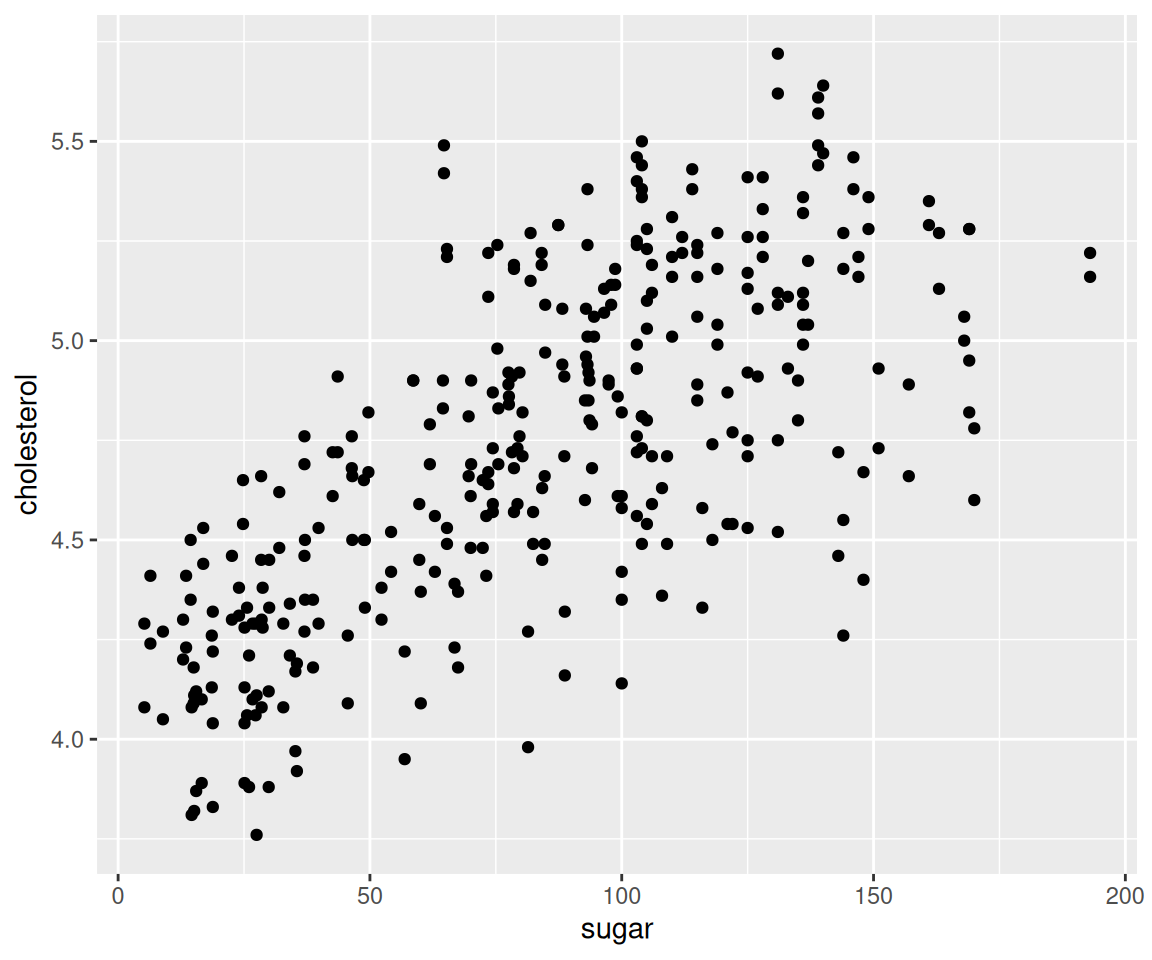To introduce the detail of the generation process of violin plot, it is helpful to review the density plot.

ggplot(data = sahp,
aes(y = sale_price)) +
geom_density()Looking at these two plots, it is easy to see that the basic violin plot is nothing but a Mirrored Density plot with the kernel density estimates on each side.

### 4.15.2 Violin plot with boxplot

Usually, the violin plot includes the boxplot inside it, providing extra information about the data. To do this, we just add the boxplot layer on top of the violin plot.

ggplot(data = sahp,
aes(x = "", y = sale_price)) +
geom_violin() +
geom_boxplot(width = 0.1)Here, we set the aesthetic width = 0.1 in the boxplot to make it thinner.

Just like in the boxplot, we can compare the distributions of a continuous variable for different values of a discrete variable. We can achieve this by mapping the discrete variable to the x axis.

ggplot(data = sahp,
aes(x = house_style, y = sale_price)) +
geom_violin() +
geom_boxplot(width = 0.1)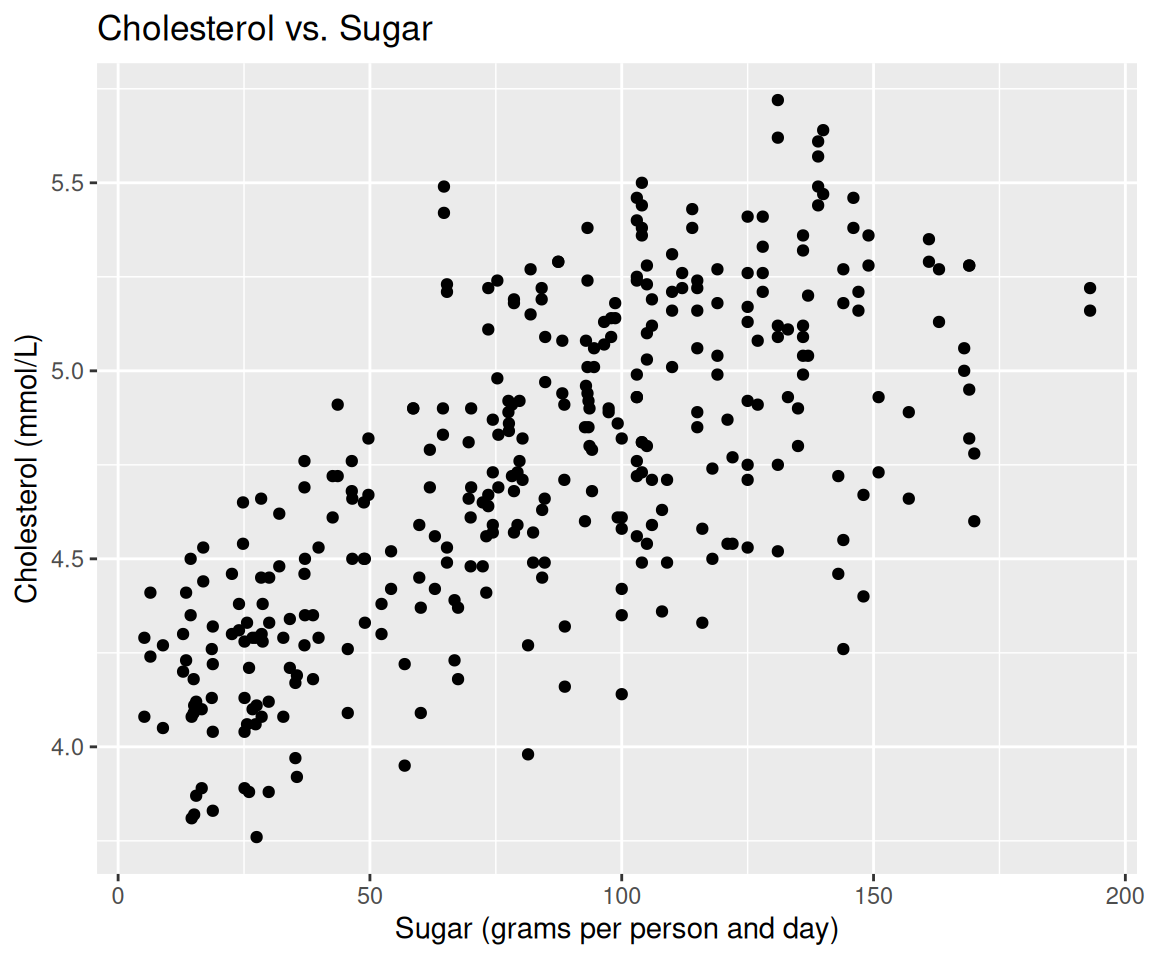We can restrict the x-axis to a subset of the possible house_style values.

ggplot(data = sahp,
aes(x = house_style,
y = sale_price,
color = house_style)) +
geom_violin() +
geom_boxplot(width = 0.1) +
scale_x_discrete(limits=c("1Story", "2Story"))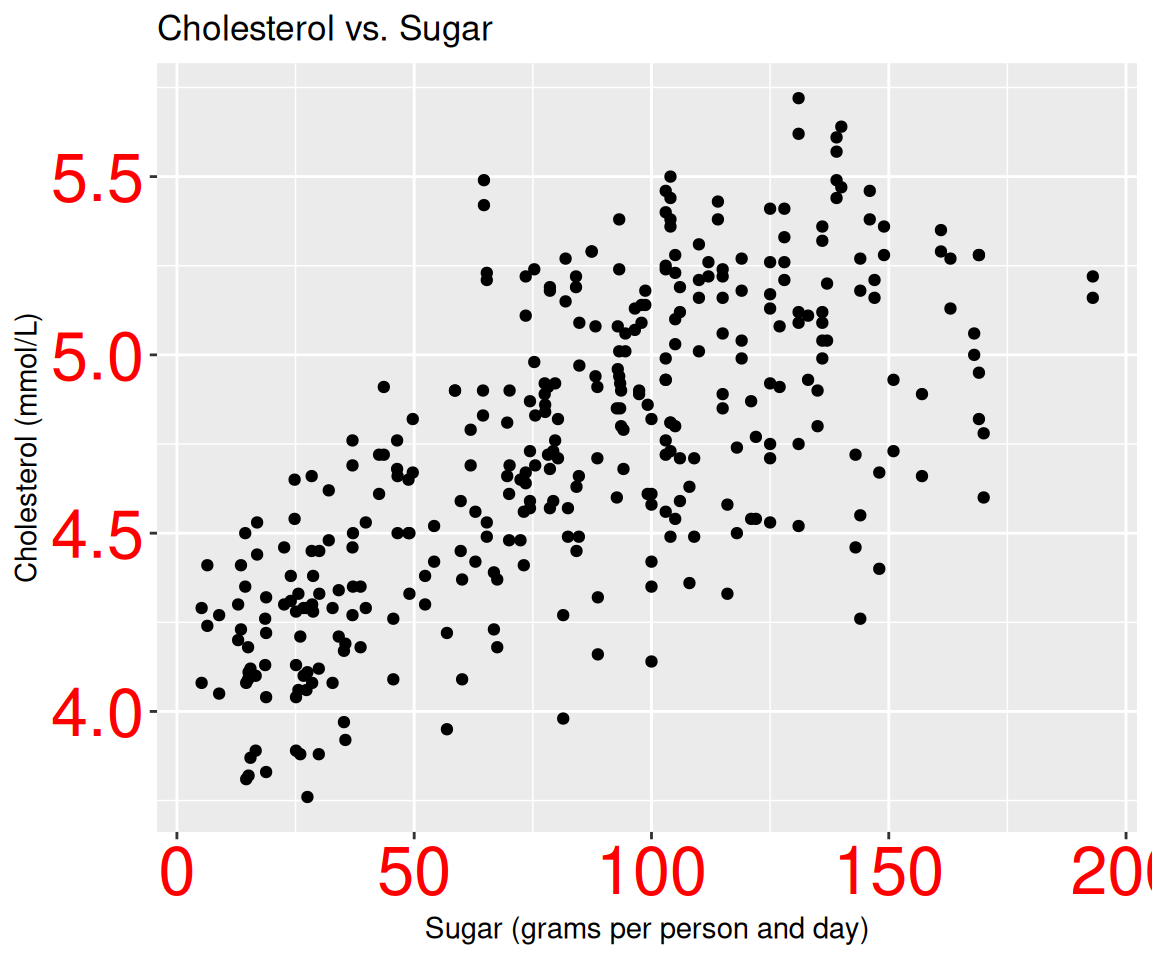Similarly, we can map a third variable to an aesthetic.

ggplot(data = remove_missing(sahp, vars="oa_qual"),
aes(x = house_style,
y = sale_price,
fill = oa_qual > 5)) +
geom_violin() +
geom_boxplot(width = 0.1) +
scale_x_discrete(limits=c("1Story", "2Story"))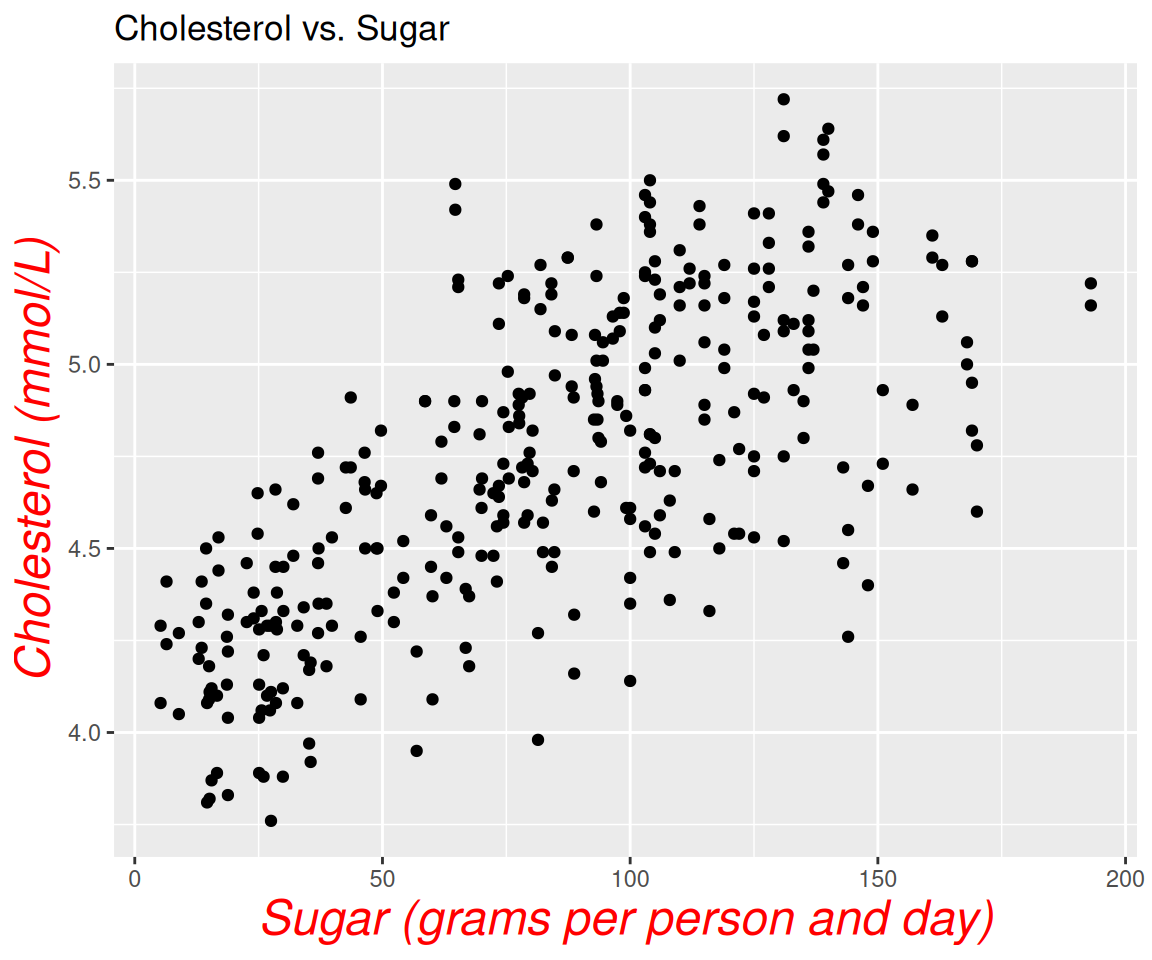As you can see, the boxplot doesn’t align well inside the violin plot. To fix this issue, you can add the global aesthetic position = position_dodge(0.9) to both geoms.

ggplot(data = remove_missing(sahp, vars="oa_qual"),
aes(x = house_style,
y = sale_price,
fill = oa_qual > 5)) +
scale_x_discrete(limits = c("1Story", "2Story")) +
geom_violin(position = position_dodge(0.9)) +
geom_boxplot(width = 0.1, position = position_dodge(0.9))You can also try to add other global aesthetics to both geoms to change their appearances.

ggplot(data = sahp,
aes(x = house_style,
y = sale_price,
fill = house_style)) +
geom_violin(color = "green", size = 2) +
geom_boxplot(width = 0.1, color = "blue", size = 1) +
scale_x_discrete(limits=c("1Story", "2Story"))